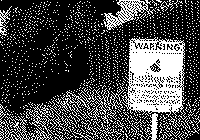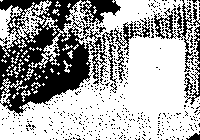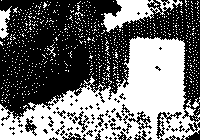# ball-morphology

Morphological operations with ball shaped structuring elements

## Usage no npm install needed!

``````<script type="module">
import ballMorphology from 'https://cdn.skypack.dev/ball-morphology';
</script>``````

# ball-morphology

Mathematical morphology for ndarrays where the structuring element is a ball in some Lp metric.

# Example

``````var morphology = require("ball-morphology")

require("get-pixels")("bwimage.png", function(err, data) {
var r = data.pick(-1, -1, 0)

// ... do stuff ...
})
``````

Which gives us the following array:We can dilate the image using the following command:

``````morphology.dilate(r, 1)
``````

Which produces the following result:Similarly, we can also perform an erosion:

``````morphology.erode(r, 1)
``````

Giving the result:For convenience, openings and closing are also implemented:

``````morphology.open(r, 1)
morphology.close(r, 1)
``````## Install

``````npm install ball-morphology
``````

## API

``````var morphology = require("ball-morphology")
``````

### `morphology.dilate(array, radius[, p])`

Performs a binary morphological dilation with an Lp ball of a given radius

• `array` is a binary image (updated in place)
• `radius` is the radius of the ball in pixel units (may be fractional)
• `p` is an optional argument giving the exponent of the metric. (Default 2)

Returns `array`

### `morphology.erode(array, radius[, p])`

Performs a binary morphological erosion with an Lp ball of a given radius

• `array` is a binary image (updated in place)
• `radius` is the radius of the ball in pixel units (may be fractional)
• `p` is an optional argument giving the exponent of the metric. (Default 2)

Returns `array`

### `morphology.open(array, radius[, p])`

Performs a binary morphological opening with an Lp ball of a given radius

• `array` is a binary image (updated in place)
• `radius` is the radius of the ball in pixel units (may be fractional)
• `p` is an optional argument giving the exponent of the metric. (Default 2)

Returns `array`

### `morphology.close(array, radius[, p])`

Performs a binary morphological closing with an Lp ball of a given radius

• `array` is a binary image (updated in place)
• `radius` is the radius of the ball in pixel units (may be fractional)
• `p` is an optional argument giving the exponent of the metric. (Default 2)

Returns `array`

# Credits

(c) 2013 Mikola Lysenko. MIT License